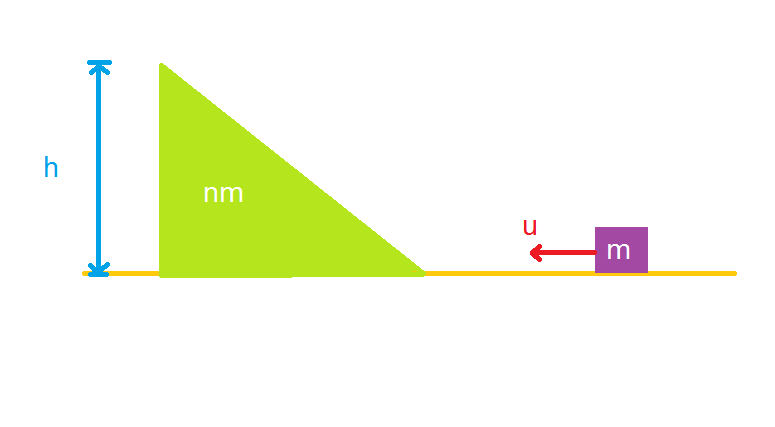Mass and WedgeA block of mass $m$ is pushed towards a movable wedge of mass $nm$ and height $h$. You may assume all the surfaces to be smooth. The minimum value of the velocity $u$ with which the block $m$ must be projected so that it would reach the top of the wedge is

×